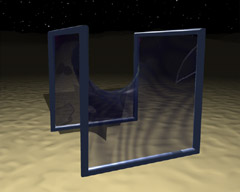# Touching Soap Films

An Introduction to Minimal Surfaces
By Hermann Karcher and Konrad Polthier

Up
Prev
Next

## Geometric Properties IIFig 8. Different assembling of the Gergonne surface of Schwarz, see Fig 6.

Now we can return to the curvature of a surface - it leads back to the curvature of a plane curve. Let us consider a fixed point on the surface. The plane that best approximates the surface in the immediate vicinity of this point is called the tangent plane, and the straight line that is perpendicular to the tangent plane at this point is called the surface normal. Every plane that contains the surface normal cuts the surface in a curve, a so-called normal section.

Since a minimal surface looks like a saddle in the neighborhood of every one of its points, some normal sections are curved toward one side of the surface and some are curved toward the other. On each side, there is a normal section with maximum curvature; as a rule the planes to which these two normal sections belong are perpendicular.

Minimal surfaces, as we have already said, are equally curved toward both sides. In other words, at every point the curvatures of the two maximal normal sections are equal but have opposite signs. This equilibrium of the curvatures is probably the basis for the aesthetic charm of minimal surfaces.

Mathematicians call a surface a minimal surface if at every one of its points it is in curvature equilibrium, and not-as its name suggests exactly-because it has the smallest possible area for a given boundary curve. This characterization has many advantages: for one, it can be examined pointwise, since the curvature at every point can be calculated, whereas for the comparison of areas the entire surface, together with surfaces that differ from it very slightly, would have to be examined. In the second place, the definition is independent of the boundary curve. Consequently, surfaces without a boundary, which extend to infinity, may also be minimal surfaces.